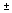# Number Sets, Infinity, and Zero

## Continue examining the number line and the relationships among sets of numbers that make up the real number system. Explore which operations and properties hold true for each of the sets. Consider the magnitude of these infinite sets and discover that infinity comes in more than one size. Examine place value and the significance of zero in a place value system.

In This Session:
Part A: Number Sets
Part B: The Size of Infinity
Part C: Examining Zero
Homework

In Session 1, you began to examine the structure of the real number system. You explored some of the elements, operations, and laws that governed this system. You then looked at the number line and discussed the concept of density. In this session, you will continue your exploration of the number sets that make up the real number system and look more closely at the concept of infinity and the importance of zero.

LEARNING OBJECTIVES
In this session, you will do the following:
• Analyze the number line and the relationships among the different sets of numbers in the real number system
• Understand different sizes of infinity
• Understand the unique qualities of zero
• Understand the behavior of a positional number system
• Understand the behavior of zero in multiplication and division
• Explore infinity and zero in the context of a graph of an equation

### Key Terms

Previously Introduced:

algebraic numbers

Algebraic numbers are all the numbers that are solutions to polynomial equations where the polynomials have rational coefficients (e.g. 1/2x3 – 3x2 + 17x + 5/8). They include all integers, rational numbers, and some irrational numbers (e.g.,, which are the solutions to x2 – 2 = 0). Note that algebraic numbers also include some complex numbers (e.g.,, the solutions to x2+ 1 = 0).

complex numbers

Complex numbers are numbers composed by the addition of imaginary and real number elements. They are in the form a + bi, where a and b are real numbers, and i can be represented as i2 = -1 (a number such that when you square it, you get -1). Graphically, the complex numbers are typically shown as a plane, where the real part is represented horizontally and the imaginary part is represented vertically. The complex numbers are uncountably infinite, are closed under the four basic operations (other than dividing by 0), and have additive and multiplicative identity and inverses (other than 0). Additionally, the complex numbers are closed under any polynomial; that is, any polynomial with complex-number coefficients will have all its roots in the complex numbers.

counting numbers

Counting numbers are the same as natural numbers (i.e., 1, 2, 3, 4, …).

integers

Integers include positive and negative whole numbers, and 0.

irrational numbers

Irrational numbers are numbers that can’t be expressed as a quotient of two integers, such as pi or square roots; they can only be expressed as infinite, non-repeating decimals.

pure imaginary numbers

Pure imaginary numbers are numbers of the form b • i, where b is a real number and i is a number such that when squared yields -1 (i.e., i2 = -1). Graphically, the pure imaginary numbers are typically shown as a vertical line intersecting the number line at 0. They are uncountably infinite, closed under addition and subtraction only, and have additive identity and inverses.

rational numbers

Rational numbers are numbers that can be expressed as a quotient of two integers; when expressed in a decimal form they will either terminate (1/2 = 0.5) or repeat (1/3 = 0.333…)

real numbers

Real numbers comprise all rational and irrational numbers. They can be represented on a number line.

transcendental numbers

Transcendental numbers are numbers that cannot be the solution to a polynomial equation. The most common transcendental numbers areand e.

whole numbers

Whole numbers are the counting numbers and zero.

New in This Session:

countably infinite set

A countably infinite set can be put into one-to-one correspondence with the counting numbers {1, 2, 3, 4, 5, …}. For example, the positive even numbers are countably infinite, since we can find a one-to-one mapping of {2, 4, 6, 8, 10, …} onto the counting numbers. Some examples of countably infinite sets are the whole numbers, integers, and rational numbers.

infinite set

An infinite set can be put into one-to-one correspondence with a proper subset of itself. For example, the counting numbers {1, 2, 3, 4, 5, …} can be put into one-to-one correspondence with the subset {2, 3, 4, 5, 6, …}, so the set must be infinite. Some examples of infinite sets are the integers and real numbers.

uncountably infinite set

An uncountably infinite set cannot be put into one-to-one correspondence with the counting numbers {1, 2, 3, 4, 5,…}. Proving that a set is uncountable is typically done indirectly, by first assuming that it is countable and then finding a contradiction. The real numbers and complex numbers are uncountably infinite.